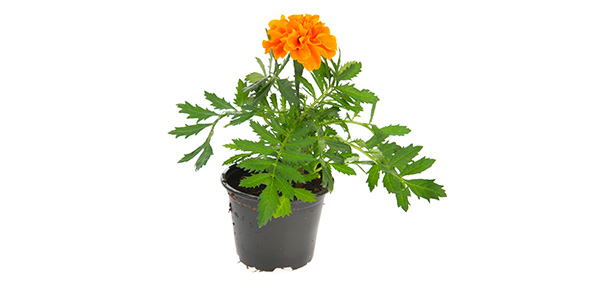# Power Plant Cpl - IR

50 Questions | Total Attempts: 103SettingsCreate your own Quiz• 1.
The principle of developing propulsion force of the jet engine is :
• A.

A.      Accelerating mass air through combustion process

• B.

B.      Pressurizing mass air through combustion process

• C.

C.      Reaction equal with action

• D.

D.      All are correct

• 2.
The main components of Gas Turbine are :
• A.

A.      Piston, Cylinder, Crankcase

• B.

B.      Compressor, Combustion chamber, Crankshaft

• C.

C.      Piston, Cylinder, Turbine

• D.

D.     Compressor, combustion chamber, Turbine

• 3.
The type of fuel used in the Gas Turbine Engine is :
• A.

A.      Avigas

• B.

B.     Kerosine base

• C.

C.      Gasoline base

• D.

D.      Methanol

• 4.
Types of Gas Turbine Engine used on aircraft is :
• A.

A.      Turbojet

• B.

B.      Turboprop / Turbo shaft

• C.

C.      Turbofan

• D.

D.     All are correct

• 5.
The function of Turbine rotor is :
• A.

A.      Converting heat energy into pressure energy

• B.

B.      Converting kinetic energy into pressure energy

• C.

C.      Converting kinetic energy into mechanical energy

• D.

D.      Converting heat energy into velocity energy

• 6.
Types compressor used on aircraft Gas Turbine Engine :
• A.

A.      Piston and type compressor

• B.

B.      Gear and screw type compressor

• C.

C.      Radial and vane type compressor

• D.

D.     Axial and centrifugal type compressor

• 7.
Where is the highest pressure of gas in the Gas Turbine Engine
• A.

A.      Inlet compressor

• B.

B.     Inlet combustion chamber

• C.

C.      Inlet turbine

• D.

D.      Exhaust duct

• 8.
What is engine component which contact to the highest gas temperature ?
• A.

A.      Compressor

• B.

B.      Combustion chamber

• C.

C.      Turbine

• D.

D.      Exhaust duct

• 9.
Where is the location of temperature probes or temperature sensors of the engine
• A.

A.      Engine inlet

• B.

B.      Combustion chamber

• C.

C.      Turbine

• D.

D.      Exhaust duct

• 10.
What is the meaning of Hush Kit ?
• A.

A.      Noise reducer

• B.

B.      Noise suppressor

• C.

C.      Noise absorber

• D.

D.     All are correct

• 11.
Where is the location of Hush Kit ?
• A.

A.      Engine inlet

• B.

B.      Combustion chamber

• C.

C.      Turbine

• D.

D.     Exhaust nozlle

• 12.
How many igniter plug at least should be installed in the combustion chamber ?
• A.

A.      1

• B.

B.     2

• C.

C.      3

• D.

D.      4

• 13.
What is the correct sequence of starting the Gas Turbine Engine ?
• A.

A.      Starter On, Ignition On and Fuel On

• B.

B.      Starter On, Fuel On and Ignition On

• C.

C.      Fuel On, Starter On and Fuel On

• D.

D.      Ignition On, Starter On and Fuel On

• 14.
What is the method of introducing fuel into combustion chamber ?
• A.

A.      Carburetor

• B.

B.      Fuel droplet

• C.

C.      Fuel sprayer

• D.

D.      Fuel gaseous

• 15.
The principle of jet propulsion is based on……………third law
• A.

A.      Pascal

• B.

B.     Newton

• C.

C.      Hero

• D.

D.      Leonardo de Vinci

• 16.
The jet engines that was called Non Air Breathing engines is:
• A.

A.    Rocket.

• B.

B.    Ramjets.

• C.

C.     Pulse jet.

• D.

D.    Turbojet.

• 17.
Compressor pressure Ratio is :
• A.

A.    The ratio of total pressure at compressor outlet to the total pressure at compressor inlet

• B.

B.    The ratio of total pressure at compressor entry to static l pressure at compressor inlet

• C.

C.     The ratio of total pressure at turbine outlet to the total pressure at compressor inlet

• D.

D.    The ratio of total pressure at turbine outlet to the total pressure at turbine inlet

• 18.
The turbine engine cycle is :
• A.

A.    Intake, Compression, combustion, Exhaust

• B.

B.    Intake, Compression, combustion, Exhaust, Recycle intake

• C.

C.     Intake, Compression, combustion, Exhaust, Re-heater

• D.

D.    Compression, combustion, Expansion.

• 19.
The Thermodynamic process during compression is:
• A.

A.    Isothermal process.

• B.

• C.

C.     Polytropic process.

• D.

D.    Isobaric process

• 20.
The propulsive force on the jet engines obtained from :
• A.

A.    Rotating propeller.

• B.

B.    High pressure air discharged from compressors.

• C.

C.     Accelerated mass of air through combustion process.

• D.

D.    Impulse reaction.

• 21.
There are two types of Turbo Fan engines :
• A.

A.    Single spool and Twin spool engines.

• B.

B.    Low bypass and High bypass engines.

• C.

C.     Multi fan and single fan.

• D.

D.    Forward fan and aft fan.

• 22.
What the percentage of the power turbine on turbo prop absorb heat energy from combustion process:
• A.

A.    20 to 25 %

• B.

B.    40 to 60%

• C.

C.     80 to 90%

• D.

D.    100%

• 23.
Which statement is not the advantage of Turbo Fan over Turbo Jet :
• A.

A.    Low noise level

• B.

B.    Low fuel consumptions.

• C.

C.    High noise level.

• D.

D.    Good performance at low and high speed.

• 24.
What the inlet duct use for subsonic aircraft :
• A.

A.    Convergent duct

• B.

B.    Divergent duct

• C.

C.     Bell mouth inlet duct

• D.

D.    Variable geometric inlet duct.

• 25.
What is mean by Thrust Specific Fuel consumption :
• A.

A.    Fuel flow multiply by Thrust.

• B.

B.    Fuel flow divided by Thrust.

• C.

C.     Fuel flow divided by Flight hours .

• D.

D.    Fuel flow divided by weight of fuel.

Related TopicsBack to top
×

Wait!
Here's an interesting quiz for you.